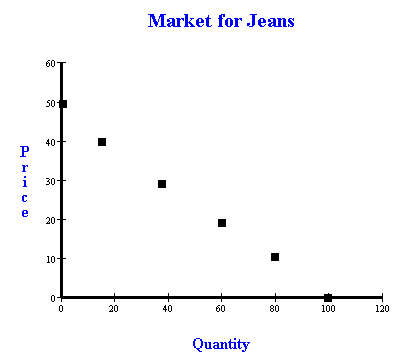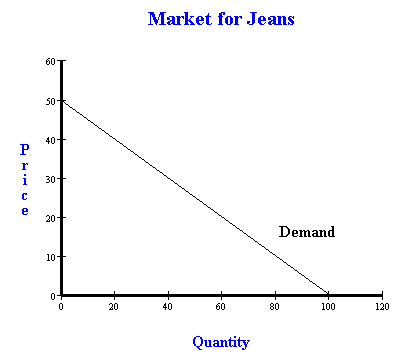Making a demand curve, understanding what a demand schedule is. - FreeEconHelp.com, Learning Economics... Solved!

## 7/2/11

In this post we will go over what a demand curve is, what it means, and how we can construct one.  I will also remind you that when constructing a demand curve remembering the letter D is important, as in D for demand, and D for down (because demand curves are always downward sloping, and if a teacher gives you a question where the demand IS upward sloping then it is a giffen good which doesn’t exist and your teacher is being frustrating).

Anyways, when constructing a demand curve you need a demand schedule.  A demand schedule shows you the quantity that will be demanded at every price level.  We can relate this to our own lives pretty easily.  If something is cheaper, we are going to buy more of it!  Think about jeans, if you see a pair of jeans you really like for \$30, you may buy one or two.  But now the same jeans are \$10, you may buy four or five.  Now consider EVERYONE in the economy.  If the price of jeans goes to \$10, then a whole lot more of them are going to be sold.  This is, in essence, what goes on behind a demand curve.

 Demand for Jeans Quantity Price 100 0 80 10 60 20 40 30 20 40 0 50

Above you see an example of a demand schedule.  It shows us how many units (quantity) of a good will be demanded at a given price.  The above schedule gives us a linear demand curve, because for every \$10 the price goes up, quantity goes down by 20.  We can use this schedule to plot the following points on a graph.Since we know that the curve is linear, we can just connect these points to get our demand curve (we could also connect the points if it wasn’t linear, it just wouldn’t be as exact).  As you can see it is actually very simple to construct a demand curve graphically if you are given the demand schedule.  You can also reverse engineer a demand schedule if you are given a demand curve as long as you can tell where the points are in the graph.That is pretty much it for the demand curve.  Remember that the difference between the demand schedule and the demand curve is just how it is presented to you, either in a table or graphical form.  The important thing to remember is that demand curves slope down (likewise supply curves slope up).  A good trick for remembering this is that demand starts with the letter D, and so does the word down.  So D is for demand, and D is also for down!  I know it seems simple, but in the heat of the exam they might get confusing, but knowing this little trick will help you out because it is so easy!  Good luck.

Alternatively, you can also derive a demand curve from a demand function. This is done by plugging in values to the demand function and creating a demand schedule as seen above. Once you have enough values you can start to plot them to make the demand curve that is associated with the demand function.